## 2017年12月30日 星期六

### Gnuplot-3D

splot "data.txt" using 1:2:3 with lines

#data.txt 每一條線隔2行空白, 線可分開繪製, 若是只隔一行空白則會連起來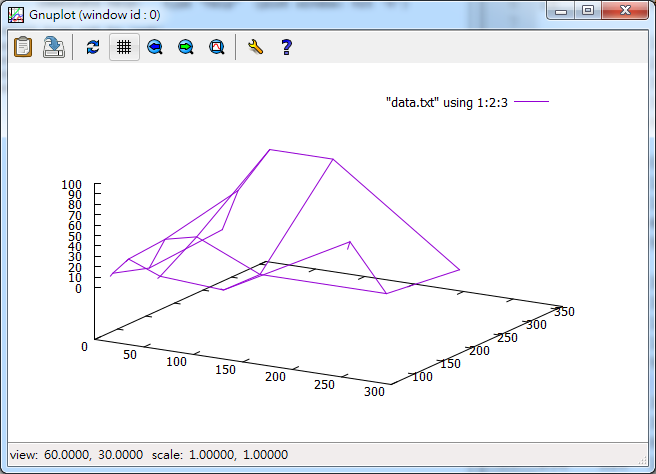#data.txt
# X   Y    Z
16    16   0
16   32   1
16   64   15
16    128  4
16    256  9

32    16   10
32   32   21
32    64   31
32    128  34
32    256  49

64    16   0
64   32   12
64   64   19
64    128  41
64    256  93

128   16   30
128   32   31
128   64   15
128   128  14
128   256  93

256   16   30
256   32   31
256   64   80
256   128  14
256   256  5

## 2017年12月7日 星期四

### GPU vs CPU

CPU: model name   : Intel(R) Xeon(R) CPU E5-2620 v3 @ 2.40GHz
RAM: 10G
OS: Ubuntu 16.04

CPU model name    : Intel Core i7-7700K CPU @ 4.5GHz
GPU: GeForce GTX 1080
RAM:  32G

## 2017年11月22日 星期三

### .py 和 ipython notebook (.ipynb) 互轉?

1) 如何將 xxx .py 轉成 xxx.ipynb ?

2) 如何將 xxx .ipynb 轉成 xxx.py ?

!jupyter nbconvert --to script hw3.ipynb

## 2017年11月17日 星期五

### CNN using keras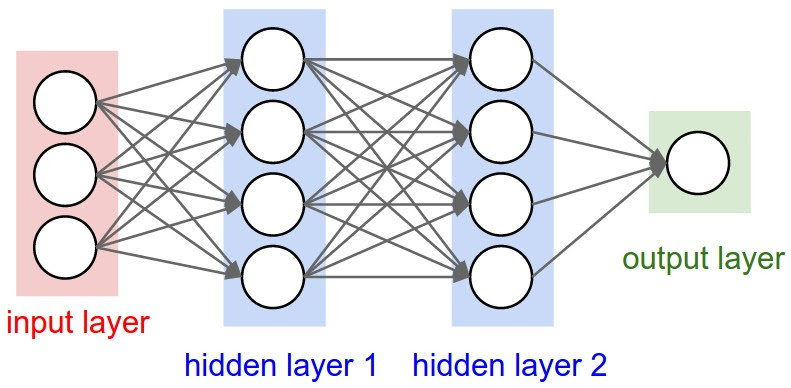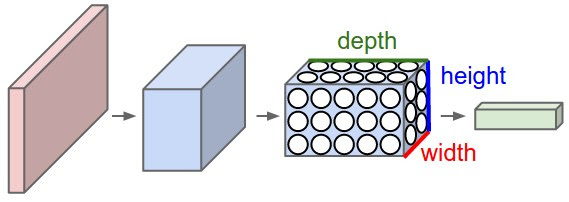A ConvNet arranges its neurons in three dimensions (width, height, depth), as visualized in one of the layers. Every layer of a ConvNet transforms the 3D input volume to a 3D output volume of neuron activations. In this example, the red input layer holds the image, so its width and height would be the dimensions of the image, and the depth would be 3 (Red, Green, Blue channels).An example input volume in red (e.g. a 32x32x3 CIFAR-10 image), and an example volume of neurons in the first Convolutional layer. Each neuron in the convolutional layer is connected only to a local region in the input volume spatially, but to the full depth (i.e. all color channels). Note, there are multiple neurons (5 in this example) along the depth, all looking at the same region in the input - see discussion of depth columns in text below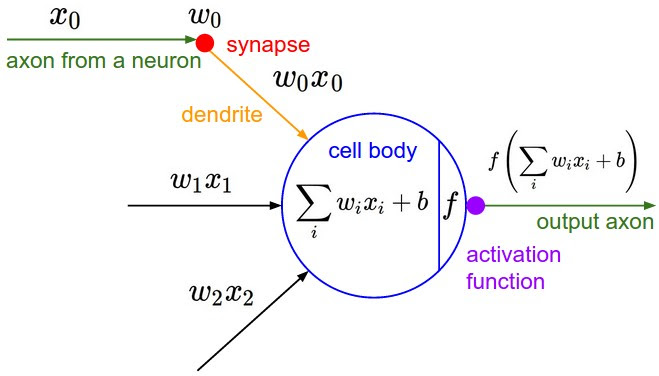he neurons from the Neural Network chapter remain unchanged: They still compute a dot product of their weights with the input followed by a non-linearity, but their connectivity is now restricted to be local spatially.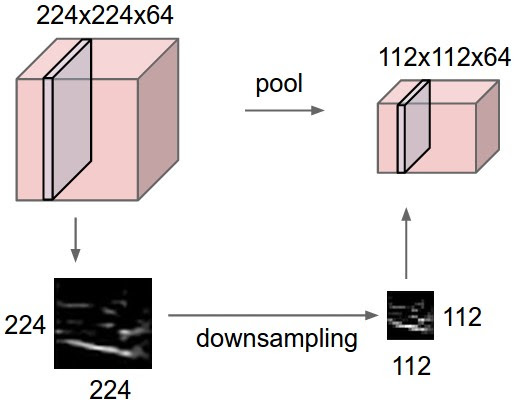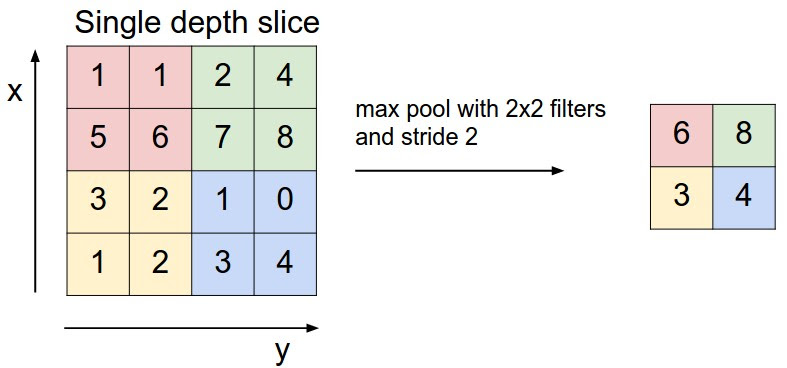Pooling layer downsamples the volume spatially, independently in each depth slice of the input volume. Left: In this example, the input volume of size [224x224x64] is pooled with filter size 2, stride 2 into output volume of size [112x112x64]. Notice that the volume depth is preserved. Right: The most common downsampling operation is max, giving rise to max pooling, here shown with a stride of 2. That is, each max is taken over 4 numbers (little 2x2 square).

# The data, shuffled and split between train and test sets:
(x_train, y_train), (x_test, y_test) = cifar10.load_data()
print('x_train shape:', x_train.shape)
print('y_train shape:', y_train.shape)
print(x_train.shape, 'train samples')
print(x_test.shape, 'test samples')

# Convert class vectors to binary class matrices.
y_train = keras.utils.to_categorical(y_train, num_classes)
y_test = keras.utils.to_categorical(y_test, num_classes)

model = Sequential()
print('input_shape:',x_train.shape[1:])

# initiate RMSprop optimizer
opt = keras.optimizers.rmsprop(lr=0.0001, decay=1e-6)

# Let's train the model using RMSprop
model.compile(loss='categorical_crossentropy',
optimizer=opt,
metrics=['accuracy'])
model.summary()

x_train = x_train.astype('float32')
x_test = x_test.astype('float32')
x_train /= 255
x_test /= 255

_________________________________________________________________
Layer (type)                 Output Shape              Param #
=================================================================
conv2d_46 (Conv2D)           (None, 32, 32, 2)         152  ((5*5*3)+1)*2=152
其他位置也都是共用這152參數,所以不是圖愈大參數愈多
+1: bias 項, WX+b

______________________________________________________________

activation_67 (Activation)   (None, 32, 32, 2)         0
_________________________________________________________________
conv2d_47 (Conv2D)           (None, 30, 30, 32)        608  ((3*3*2)+1)*32=608      
_________________________________________________________________
activation_68 (Activation)   (None, 30, 30, 32)        0
_________________________________________________________________
max_pooling2d_23 (MaxPooling (None, 15, 15, 32)        0
_________________________________________________________________
dropout_34 (Dropout)         (None, 15, 15, 32)        0
_________________________________________________________________
conv2d_48 (Conv2D)           (None, 15, 15, 64)        18496
_________________________________________________________________
activation_69 (Activation)   (None, 15, 15, 64)        0
_________________________________________________________________
conv2d_49 (Conv2D)           (None, 13, 13, 64)        36928
_________________________________________________________________
activation_70 (Activation)   (None, 13, 13, 64)        0
_________________________________________________________________
max_pooling2d_24 (MaxPooling (None, 6, 6, 64)          0
_________________________________________________________________
dropout_35 (Dropout)         (None, 6, 6, 64)          0
_________________________________________________________________
flatten_12 (Flatten)         (None, 2304)              0
_________________________________________________________________
dense_23 (Dense)             (None, 512)               1180160
_________________________________________________________________
activation_71 (Activation)   (None, 512)               0
_________________________________________________________________
dropout_36 (Dropout)         (None, 512)               0
_________________________________________________________________
dense_24 (Dense)             (None, 10)                5130
_________________________________________________________________
activation_72 (Activation)   (None, 10)                0
=================================================================
Total params: 1,241,474
Trainable params: 1,241,474
Non-trainable params: 0
_________________________________________________________________

## 2017年11月11日 星期六

### PCA

In PCA, we are interested to find the directions (components) that maximize the variance in our dataset. In DataSet, 某些Feature對於每一筆X有比較大的差異, 對Y分類影響會比較明顯。若Feature 對於所有X都差不多, 則此Feature並不是作為分群(Partition)的最好選擇。

PCA(n_components=2), 會自動找出前2個主要Feature。下圖為2維, 畫出其分類(0,1,2)的狀態

==============

Residual variance ?

Then you fit a regression model. You use the regression equation to calculate a predicted score for each person.

Then you find the difference between the predicted scores and the actual scores. You calculate the variance of the set of scores. It's the residual variance. The residual variance will be less than the total variance (or if your predictors are completely useless, they will be equal).

How much variance did you explain?
Explained variance = (total variance - residual variance)

The proportion of variance explained is therefore:

explained variance / total variance

If your predicted scores exactly match the outcome scores, you've perfectly predicted the scores, and you've explained all of the variance. The residuals are all zero.

(Note: The calculations are done with sums of squares, variances will give a very slightly different answer as they are usually calculated as SS/(N-1). But if the sample is large, this difference is trivial).

## 2017年11月10日 星期五

### k-fold cross-validation

#另一種方法做cross validation, 若validation data從training data拿,則training sample 就會少了..
#使用 k-fold cross-validation 可以解決此問題
scores=cross_val_score(dct, Xtrain, ytrain, cv=5)
print(scores.mean())

However, by partitioning the available data into three sets, we drastically reduce the number of samples which can be used for learning the model, and the results can depend on a particular random choice for the pair of (train, validation) sets.
A solution to this problem is a procedure called cross-validation (CV for short). A test set should still be held out for final evaluation, but the validation set is no longer needed when doing CV. In the basic approach, called k-fold CV, the training set is split into k smaller sets (other approaches are described below, but generally follow the same principles). The following procedure is followed for each of the k “folds”:
• A model is trained usingof the folds as training data;
• the resulting model is validated on the remaining part of the data (i.e., it is used as a test set to compute a performance measure such as accuracy).
The performance measure reported by k-fold cross-validation is then the average of the values computed in the loop. This approach can be computationally expensive, but does not waste too much data (as it is the case when fixing an arbitrary test set), which is a major advantage in problem such as inverse inference where the number of samples is very small.

## 2017年11月9日 星期四

### Multi-layer Perceptron classifier

sklearn.linear_model.LogisticRegression
This model optimizes the log-loss function using LBFGS or stochastic gradient descent.

solver : 要使用那一種演算法去解最佳化的問題, 如 weight optimization.

solver : {‘newton-cg’, ‘lbfgs’, ‘liblinear’, ‘sag’, ‘saga’},
default: ‘liblinear’ Algorithm to use in the optimization problem.

batch_size : int, optional, default ‘auto’
Size of minibatches for stochastic optimizers. If the solver is ‘lbfgs’, the classifier will not use minibatch. When set to “auto”, batch_size=min(200, n_samples)

max_iter : int, optional, default 200
Maximum number of iterations. The solver iterates until convergence (determined by ‘tol’) or this number of iterations. For stochastic solvers (‘sgd’, ‘adam’), note that this determines the number of epochs (how many times each data point will be used), not the number of gradient steps.
that is , total parameter update= (# of batch_size) * (# of epochs)

tol : float, optional, default 1e-4
Tolerance for the optimization. When the loss or score is not improving by at least tol for two consecutive iterations, unless learning_rate is set to ‘adaptive’, convergence is considered to be reached and training stops.
momentum : float, default 0.9
Momentum for gradient descent update. Should be between 0 and 1. Only used when solver=’sgd’.
early_stopping : bool, default False
Whether to use early stopping to terminate training when validation score is not improving. If set to true, it will automatically set aside 10% of training data as validation and terminate training when validation score is not improving by at least tol for two consecutive epochs. Only effective when solver=’sgd’ or ‘adam’
validation_fraction : float, optional, default 0.1
The proportion of training data to set aside as validation set for early stopping. Must be between 0 and 1. Only used if early_stopping is True

 fit(X, y) Fit the model to data matrix X and target(s) y. get_params([deep]) Get parameters for this estimator. predict(X) Predict using the multi-layer perceptron classifier predict_log_proba(X) Return the log of probability estimates. predict_proba(X) Probability estimates. score(X, y[, sample_weight]) Returns the mean accuracy on the given test data and labels. set_params(**params) Set the parameters of this estimator.

predict_proba(X) Probability estimates. The returned estimates for all classes are ordered by the label of classes.

Y=predict_proba(X) is a method of a (soft) classifier outputting the probability of the instance being in each of the classes.
Y 是一個list, 列出X 會落在Y分類中的機率值

## 2017年11月1日 星期三

### 資料的4種(變數)型態與 MEASUREMENT SCALES

Nominal

Let’s start with the easiest one to understand.  Nominal scales are used for labeling variables, without any quantitative value.  “Nominal” scales could simply be called “labels.”

Ordinal
Ordinal scales are typically measures of non-numeric concepts like satisfaction, happiness, discomfort, etc.

“Ordinal” is easy to remember because is sounds like “order” and that’s the key to remember with “ordinal scales”–it is the order that matters, but that’s all you really get from these.
Interval ==> +, 1
Like the others, you can remember the key points of an “interval scale” pretty easily.  “Interval” itself means “space in between,” which is the important thing to remember–interval scales not only tell us about order, but also about the value between each item.

Ratio   ==> +, - x /

Ratio scales provide a wealth of possibilities when it comes to statistical analysis.

Summary
In summary, nominal variables are used to “name,” or label a series of values.  Ordinal scales provide good information about the order of choices, such as in a customer satisfaction survey.  Interval scales give us the order of values + the ability to quantify the difference between each one.  Finally, Ratio scales give us the ultimate–order, interval values, plus the ability to calculate ratios since a “true zero” can be defined.

### Multi class classification轉成binary classification 的問題

Multi class classification 轉成binary classification 的問題

Multiclass option can be either ‘ovr’ or ‘multinomial’. If the option chosen is ‘ovr’, then a binary problem is fit for each label. Else the loss minimised is the multinomial loss fit across the entire probability distribution. Does not work for liblinear solver.